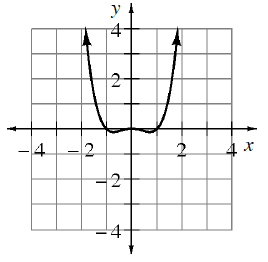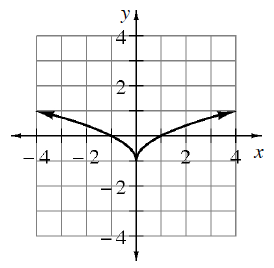### Home > APCALC > Chapter 5 > Lesson 5.1.3 > Problem5-25

5-25.

Use the graphs of $y=f^{\prime} ( x )$ and $y=g^{\prime} ( x )$ below to determine the $x$-values of all local minima, maxima, and points of inflection.

1.  $f^{\prime} ( x )$$f ( x )$ will be increasing where $f^{\prime} ( x )$ is positive, and vice versa.
$f ( x )$ will be concave up where $f^{\prime} ( x )$ has positive slopes, and vice versa.

Local max at $x = −1$; $f^{\prime} ( x )$ changes from positive to negative.
Inflection points at $x = −0.5, 0, 0.5$; $f^{\prime} ( x )$ changes slope.
Local min at $x = 1; f^\prime\left(x\right)$ changes from negative to positive.

1.  $g^{\prime} (x)$See the hint in part (a).